# GPU Particle Animation w/ Simplex Noise

11,661 total views,  1 views today

In the previous tutorial (Intro to Custom Particle Vertex Streams) we learned how to make a basic particle shader for reading and using vertex streams sent from a properly configured particle system. The end result was some bouncy-looking spring particle effect that didn’t do anything too creative, or look all that impressive. In this tutorial, we’re going to take it to the next level and make this:

[IMAGE REMOVED]

Believe it or not, if you’ve already gone through the previous tutorial (which you should!) and have the script from the 3D Uniform Particle Grid tutorial handy (optional), the effect we’ll be making in this tutorial is very easy. All we’re really doing now is passing an additional 3D vertex stream for each particle’s center and then using that to determine the vertex offset from a noise function. In this case, Simplex noise (which is similar to Perlin noise).

## Part 1 – Particle System Setup

We’ll start off by first setting up a basic particle system we can use to test the effect on. We’ll be using the default particle texture, and the final result will look something like this (with post-processing).

[IMAGE REMOVED]

Create a new particle system!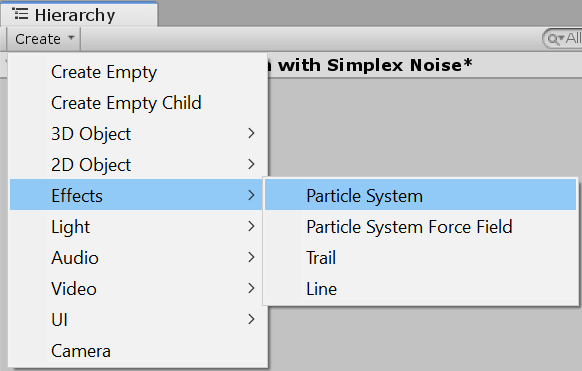Make sure to reset its Transform, and then copy the following settings for the Main module. The changes I made were enabling Prewarm, setting Start Speed to 0, randomizing Start Size to between 0.25 and 1, and setting the Max Particles to 10,000.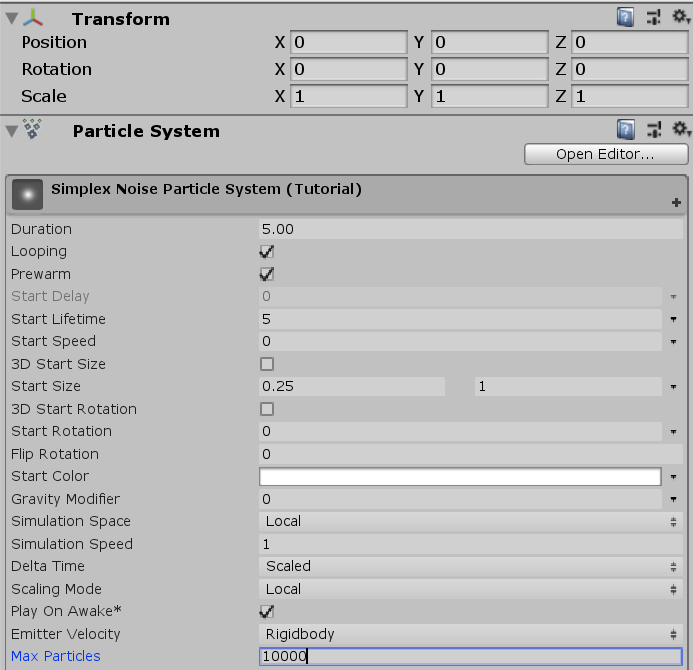Set the Emission module’s Rate over Time to 2,000.Change the Shape to Box, and the Scale to [50, 0, 50].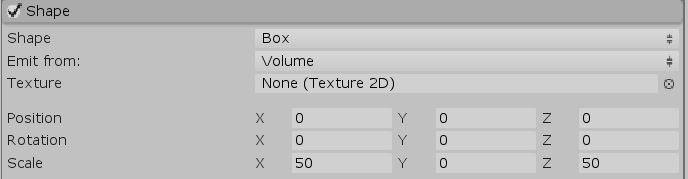You should have now have a basic flat bed of particles popping in and out.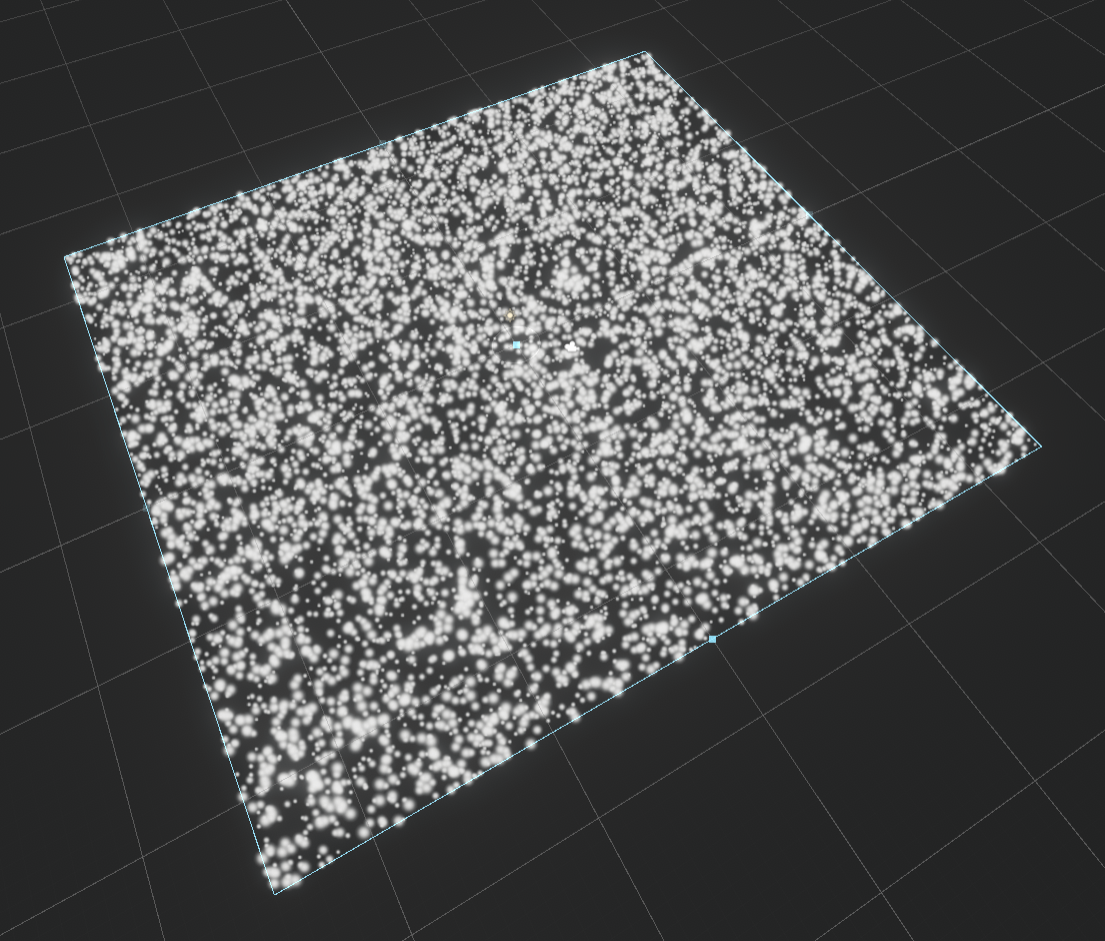We need to smooth out the spawning, so let’s enable the Color over Lifetime module. Set the gradient to something like this to fade the particles in/out.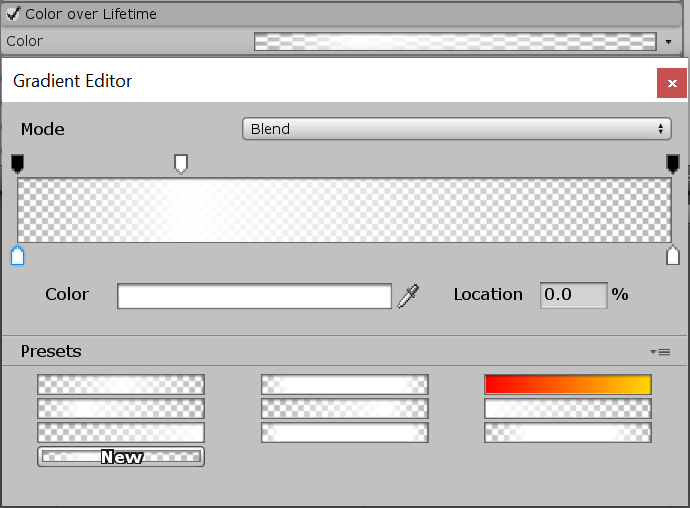Finally, in the Renderer module, enable Custom Vertex Streams and add Center.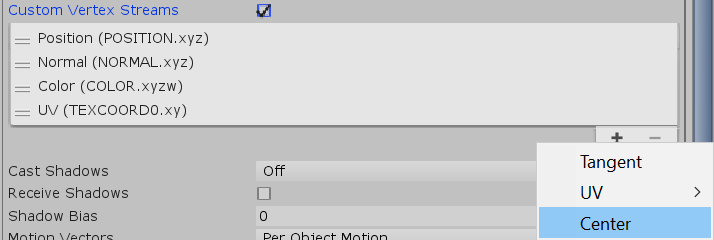This causes an overflow into TEXCOORD1 which we’ll handle once we work on our shader.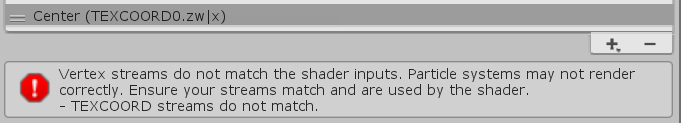And that’s it for the test particle system. Next up, our shader!

## Part 2 – Base Shader

In this part we’ll create the baseline shader needed to handle the additional vertex stream data. It’ll be based off the basic unlit particle shader we created in the last tutorial, so I’ll paste that here for reference without any of the previous tutorial-specific code. You’ll notice I’ve changed uv to tc0, as I think it’s less confusing moving forward.

```Shader "Unlit/Simple Particle Unlit"
{
Properties
{
_MainTex("Texture", 2D) = "white" {}
}

{
Tags { "Queue" = "Transparent" "RenderType" = "Opaque" }
LOD 100

Blend One One // Additive blending.
ZWrite Off // Depth test off.

Pass
{
CGPROGRAM
#pragma vertex vert
#pragma fragment frag
// make fog work
#pragma multi_compile_fog

#include "UnityCG.cginc"

struct appdata
{
float4 vertex : POSITION;
fixed4 color : COLOR;
float3 tc0 : TEXCOORD0;
};

struct v2f
{
float3 tc0 : TEXCOORD0;
UNITY_FOG_COORDS(1)
float4 vertex : SV_POSITION;
fixed4 color : COLOR;
};

sampler2D _MainTex;
float4 _MainTex_ST;

v2f vert(appdata v)
{
v2f o;

float3 vertexOffset = 0;

v.vertex.xyz += vertexOffset;
o.vertex = UnityObjectToClipPos(v.vertex);

// Initialize outgoing colour with the data recieved from the particle system stored in the colour vertex input.

o.color = v.color;

o.tc0.xy = TRANSFORM_TEX(v.tc0, _MainTex);

// Initialize outgoing tex coord variables.

o.tc0.z = v.tc0.z;

UNITY_TRANSFER_FOG(o,o.vertex);
return o;
}

fixed4 frag(v2f i) : SV_Target
{
// sample the texture
fixed4 col = tex2D(_MainTex, i.tc0);

// Multiply texture colour with the particle system's vertex colour input.

col *= i.color;
col *= col.a;

// apply fog
UNITY_APPLY_FOG(i.fogCoord, col);
return col;
}
ENDCG
}
}
}
```

We need to start making a few changes, starting with the name.

```Shader "Unlit/Simplex Noise Particle Unlit"
```

I’ve made tc0 (formerly “uv”) a float4, and added TEXCOORD1 as tc1. This’ll allow us to pass in and hold more custom vertex stream data from the particle system using this shader.

```			struct appdata
{
float4 vertex : POSITION;
fixed4 color : COLOR;
float4 tc0 : TEXCOORD0;
float4 tc1 : TEXCOORD1;
};

struct v2f
{
float4 tc0 : TEXCOORD0;
float4 tc1 : TEXCOORD1;
UNITY_FOG_COORDS(1)
float4 vertex : SV_POSITION;
fixed4 color : COLOR;
};
```

Next, I’ve initialized the outgoing tc0.zw to whatever is in the input tc0.zw (line 18). I only assign z and w since xy are already assigned as the texture’s UV coordinates (line 14). I can dump the entirety of input tc1 to the output tc1 since it isn’t shared by any previous operation in the vertex program (line 19).

```			v2f vert (appdata v)
{
v2f o;

float3 vertexOffset = 0;

v.vertex.xyz += vertexOffset;
o.vertex = UnityObjectToClipPos(v.vertex);

// Initialize outgoing colour with the data recieved from the particle system stored in the colour vertex input.

o.color = v.color;

o.tc0.xy = TRANSFORM_TEX(v.tc0, _MainTex);

// Initialize outgoing tex coord variables.

o.tc0.zw = v.tc0.zw;
o.tc1 = v.tc1;

UNITY_TRANSFER_FOG(o,o.vertex);
return o;
}
```

We can now use this shader with the particle system we worked on in the last part without seeing that nasty warning about a stream mismatch. Head back to editor and create a new material, assign it this shader, and then set the material on the particle system’s Renderer module. Make sure to assign Unity’s default particle texture.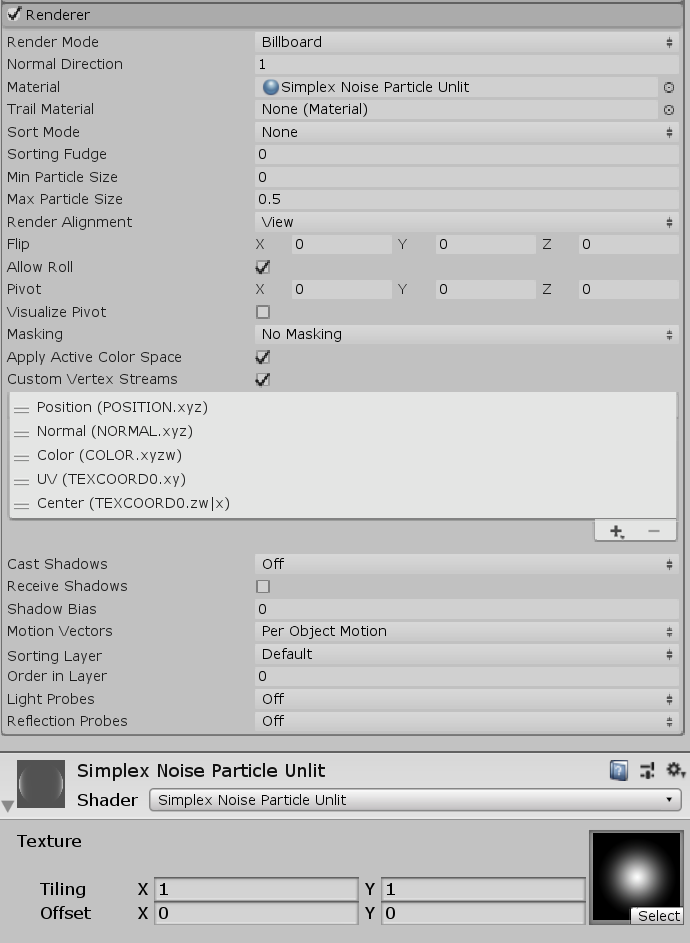If everything went well, you shouldn’t see any warnings, and your particle system should be rendering similar to how it was before (minus any fancy Standard particle shader effects).

```Shader "Unlit/Simplex Noise Particle Unlit"
{
Properties
{
_MainTex ("Texture", 2D) = "white" {}
}

{
Tags { "Queue" = "Transparent" "RenderType" = "Opaque" }
LOD 100

Blend One One // Additive blending.
ZWrite Off // Depth test off.

Pass
{
CGPROGRAM
#pragma vertex vert
#pragma fragment frag
// make fog work
#pragma multi_compile_fog

#include "UnityCG.cginc"

struct appdata
{
float4 vertex : POSITION;
fixed4 color : COLOR;
float4 tc0 : TEXCOORD0;
float4 tc1 : TEXCOORD1;
};

struct v2f
{
float4 tc0 : TEXCOORD0;
float4 tc1 : TEXCOORD1;
UNITY_FOG_COORDS(1)
float4 vertex : SV_POSITION;
fixed4 color : COLOR;
};

sampler2D _MainTex;
float4 _MainTex_ST;

v2f vert (appdata v)
{
v2f o;

float3 vertexOffset = 0;

v.vertex.xyz += vertexOffset;
o.vertex = UnityObjectToClipPos(v.vertex);

// Initialize outgoing colour with the data recieved from the particle system stored in the colour vertex input.

o.color = v.color;

o.tc0.xy = TRANSFORM_TEX(v.tc0, _MainTex);

// Initialize outgoing tex coord variables.

o.tc0.zw = v.tc0.zw;
o.tc1 = v.tc1;

UNITY_TRANSFER_FOG(o,o.vertex);
return o;
}

fixed4 frag (v2f i) : SV_Target
{
// sample the texture
fixed4 col = tex2D(_MainTex, i.tc0);

// Multiply texture colour with the particle system's vertex colour input.

col *= i.color;
col *= col.a;

// apply fog
UNITY_APPLY_FOG(i.fogCoord, col);
return col;
}
ENDCG
}
}
}
```

## Part 3 – Simplex Noise (Vertex Animation)

Here we go, the juicy bit!

We’re going to be implenting Simplex noise using Keijiro Takahashi’s HLSL implementation/translation for Unity. You can download the entire library here, which includes a few different noise functions, but we only really want/need 3D Simplex noise (SimplexNoise3D.hlsl).

SimplexNoise3D.hlsl

To be able to use the noise function inside the shader without copying the code over, we need to include it, like this:

```			#include "UnityCG.cginc"
#include "SimplexNoise3D.hlsl"
```

We’ll be adding in a few parameters to be able to control the noise speed (scrolling the offset in 3D), frequency, amplitude, and negative range clamping. Let’s add them to the shader as properties.

```		_MainTex ("Texture", 2D) = "white" {}

_NoiseSpeedX("Noise Speed X", Range(0 , 100)) = 0.0
_NoiseSpeedY("Noise Speed Y", Range(0 , 100)) = 0.0
_NoiseSpeedZ("Noise Speed Z", Range(0 , 100)) = 1.0

_NoiseFrequency("Noise Frequency", Range(0 , 1)) = 0.1
_NoiseAmplitude("Noise Amplitude", Range(0 , 10)) = 2.0

_NoiseAbs("Noise Abs", Range(0 , 1)) = 1.0
```

```			sampler2D _MainTex;
float4 _MainTex_ST;

float _NoiseSpeedX;
float _NoiseSpeedY;
float _NoiseSpeedZ;

float _NoiseFrequency;
float _NoiseAmplitude;

float _NoiseAbs;
```

Now we can get to work inside the vertex part (right before we add the offset) to actually animate the particles. Remember that the particle’s center vector is mixed in TEXCOORD0 and TEXCOORD1.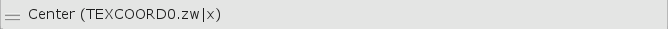This translates to the center X and Y being in tc0.zw and the Z being in tc0.x.

You may be wondering why we passed in the Center vertex stream if we’re just going to offset the vertices again like we did in the previous tutorial (where we didn’t need the per-particle center). This is because the particle vertices will be initially offset based on the particle’s world space position, which will then be as the input to the noise function. With many particles spread accross a sufficiently large area, we can see the noise distribution smoothly and animate it over time.

We can’t simply pass in the vertex position because a particle is a polygon with at least three vertices, with each one being a different point in space (read: it’s at least a triangle). If we did use the vertices, then we’d get different offsets per-vertex and you’d have some very screwy particles as the mesh itself deformed. This is not what we want! We only want the entire particle itself to move, and that means moving all of its vertices together with the same offset.

So… that’s why we use the particle’s center.

```				float3 particleCenter = float3(v.tc0.zw, v.tc1.x);
```

We can calculate the 3D noise offset (which will be added to the particle center position when passed to the Simplex function) as time * speedZ. We don’t want the noise evolving on X or Y, although we could have added invidiual noise speeds for all the axes.

```				float3 noiseOffset = _Time.y * float3(_NoiseSpeedX, _NoiseSpeedY, _NoiseSpeedZ);
```

Now we can calculate the single-dimension noise value. We initially pass in particleCenter, add the offset, then multiply the result by the frequency property.

```				float noise = snoise((particleCenter + noiseOffset) * _NoiseFrequency);
```

The snoise function gives us a value in the range [-1.0, 1.0]. Because we may want to remap this to [0.0, 1.0] and mix between the ranges, we can add in some code to do that for us by first remapping, then mixing using lerp.

```				float noise01 = (noise + 1.0) / 2.0;
float noiseRemap = lerp(noise, noise01, _NoiseAbs);
```

The vertex offset is then simply noiseRemap * _NoiseAmplitude in world space Y.

```				float3 vertexOffset = float3(0.0, noiseRemap * _NoiseAmplitude, 0.0);
v.vertex.xyz += vertexOffset;
```

That’s it for the vertex animation!

Head on back over to Unity to check out the results. Here’s an example of what you can get simply by wheeling through the particle system Start Color from its Main module.

[IMAGE REMOVED]

You can stop here if you want, but in the next part I’ll be animating the colours based on the noise value.

## Part 4 – Simplex Noise (Fragment/Pixel Colour Animation)

Since we already have our code to animate the vertices based on noise, this part is mostly done already. Let’s start off by first adding in two additional properties and global variables for the colours we’ll interpolate between based on the remapped [0.0, 1.0] noise value.

The material properties will go at the top of the shader file, right below where the previous noise properties we added. The HDR tag ensures we can pump up the intensity into the HDR range, allowing post-process effects like bloom to work well with our shader.

```		[HDR] _ColourA("Color A", Color) = (0,0,0,0)
[HDR] _ColourB("Color B", Color) = (1,1,1,1)
```

And the shader variables will go immediately below the noise variables.

```			float4 _ColourA;
float4 _ColourB;
```

Finally, in the fragment part, between when we multiply col by the input vertex colour and when we multiply col by the combined alpha, add this code:

```				col *= i.color;

float3 particleCenter = float3(i.tc0.zw, i.tc1.x);
float3 noiseOffset = _Time.y * float3(_NoiseSpeedX, _NoiseSpeedY, _NoiseSpeedZ);

float noise = snoise((particleCenter + noiseOffset) * _NoiseFrequency);
float noise01 = (noise + 1.0) / 2.0;

col = lerp(col * _ColourA, col * _ColourB, noise01);

col *= col.a;
```

You’ll notice that most of the code is copy-pasted from the vertex part, save for using “i.tc0” and “i.tc1” to get the streams from the input (line 3), and the use of lerp to interpolate between the colours (line 9).

It’s mostly self-explanatory as it only slightly modifies the code from the vertex animation part. Here’s what you should be able to do now!

[IMAGE REMOVED]

That’s everything for the shader! You can certainly stop here if you know what you’d like to do moving forward, but in the next part we’ll recreate the preview image using everything we have. Below you’ll find the full shader code.

```Shader "Unlit/Simplex Noise Particle Unlit"
{
Properties
{
_MainTex ("Texture", 2D) = "white" {}

_NoiseSpeedX("Noise Speed X", Range(0 , 100)) = 0.0
_NoiseSpeedY("Noise Speed Y", Range(0 , 100)) = 0.0
_NoiseSpeedZ("Noise Speed Z", Range(0 , 100)) = 1.0

_NoiseFrequency("Noise Frequency", Range(0 , 1)) = 0.1
_NoiseAmplitude("Noise Amplitude", Range(0 , 10)) = 2.0

_NoiseAbs("Noise Abs", Range(0 , 1)) = 1.0

[HDR] _ColourA("Color A", Color) = (0,0,0,0)
[HDR] _ColourB("Color B", Color) = (1,1,1,1)
}

{
Tags { "Queue" = "Transparent" "RenderType" = "Opaque" }
LOD 100

Blend One One // Additive blending.
ZWrite Off // Depth test off.

Pass
{
CGPROGRAM
#pragma vertex vert
#pragma fragment frag
// make fog work
#pragma multi_compile_fog

#include "UnityCG.cginc"
#include "SimplexNoise3D.hlsl"

struct appdata
{
float4 vertex : POSITION;
fixed4 color : COLOR;
float4 tc0 : TEXCOORD0;
float4 tc1 : TEXCOORD1;
};

struct v2f
{
float4 tc0 : TEXCOORD0;
float4 tc1 : TEXCOORD1;
UNITY_FOG_COORDS(1)
float4 vertex : SV_POSITION;
fixed4 color : COLOR;
};

sampler2D _MainTex;
float4 _MainTex_ST;

float _NoiseSpeedX;
float _NoiseSpeedY;
float _NoiseSpeedZ;

float _NoiseFrequency;
float _NoiseAmplitude;

float _NoiseAbs;

float4 _ColourA;
float4 _ColourB;

v2f vert (appdata v)
{
v2f o;

float3 particleCenter = float3(v.tc0.zw, v.tc1.x);
float3 noiseOffset = _Time.y * float3(_NoiseSpeedX, _NoiseSpeedY, _NoiseSpeedZ);

float noise = snoise((particleCenter + noiseOffset) * _NoiseFrequency);

float noise01 = (noise + 1.0) / 2.0;
float noiseRemap = lerp(noise, noise01, _NoiseAbs);

float3 vertexOffset = float3(0.0, noiseRemap * _NoiseAmplitude, 0.0);

v.vertex.xyz += vertexOffset;
o.vertex = UnityObjectToClipPos(v.vertex);

// Initialize outgoing colour with the data recieved from the particle system stored in the colour vertex input.

o.color = v.color;

o.tc0.xy = TRANSFORM_TEX(v.tc0, _MainTex);

// Initialize outgoing tex coord variables.

o.tc0.zw = v.tc0.zw;
o.tc1 = v.tc1;

UNITY_TRANSFER_FOG(o,o.vertex);
return o;
}

fixed4 frag (v2f i) : SV_Target
{
// sample the texture
fixed4 col = tex2D(_MainTex, i.tc0);

// Multiply texture colour with the particle system's vertex colour input.

col *= i.color;

float3 particleCenter = float3(i.tc0.zw, i.tc1.x);
float3 noiseOffset = _Time.y * float3(_NoiseSpeedX, _NoiseSpeedY, _NoiseSpeedZ);

float noise = snoise((particleCenter + noiseOffset) * _NoiseFrequency);
float noise01 = (noise + 1.0) / 2.0;

col = lerp(col * _ColourA, col * _ColourB, noise01);

col *= col.a;

// apply fog
UNITY_APPLY_FOG(i.fogCoord, col);
return col;
}
ENDCG
}
}
}
```

## Part 5 – Putting it all Together

For this last part, you’ll need to have the script from the 3D Uniform Particle Grid tutorial. We’re going to create three particle systems, each with a different material with different settings, and the same texture.

All three will have the Particle Grid script attached with the following settings: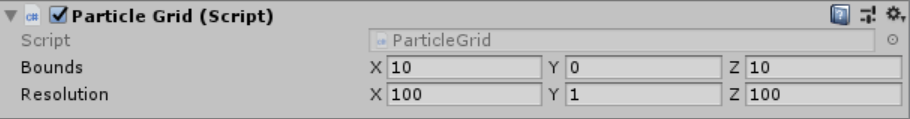All modules except for Renderer should be disabled. The emission, colour, and shape will be controlled by the script we attached.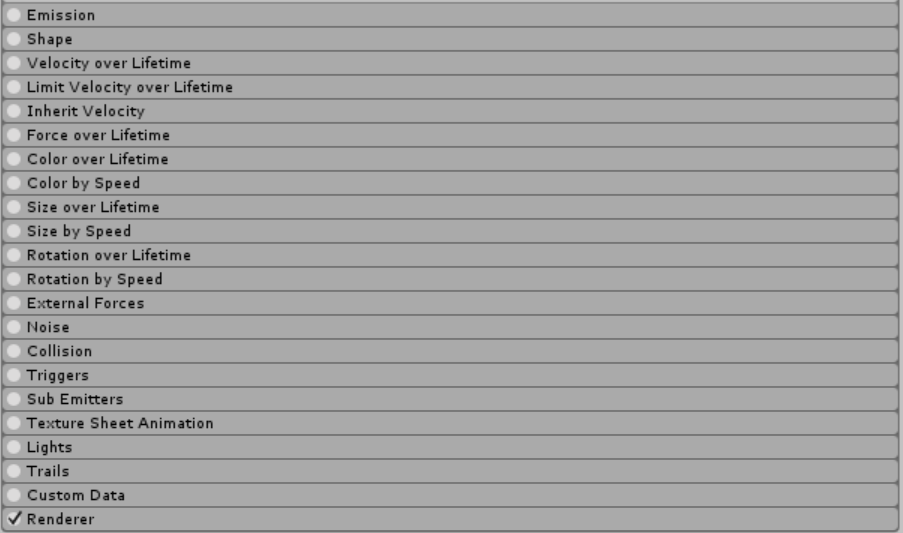All particle systems should also have these settings for the Main module. Namely, Start Lifetime is 1,000, Start Speed is 0, Start Size is 0.5, and Max Particles is set to 100,000.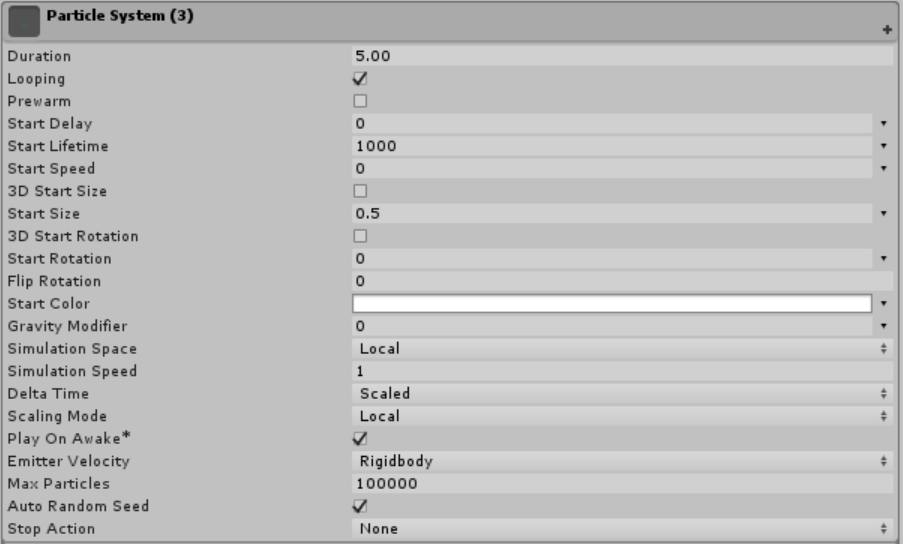Make sure to have enabled Custom Vertex Streams and added in the Center stream.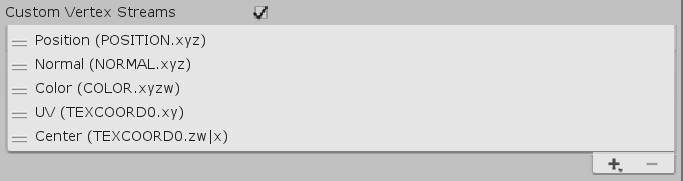### Particle System 1

This will be the green(-ish) down-scrolling grid backdrop.

[IMAGE REMOVED]

The Transform setup is shown below. Position Z = 10, Rotation X = 110, Scale = 2, 1, 1.5.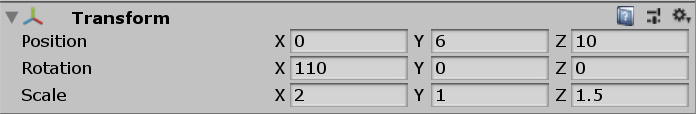Noise XYZ should be (0, 1, 0), Frequency should be 0.25, Amplitude should be 0.75, and the Abs should be 1. The two colours are between blue (alpha = 10) and green (alpha = 75).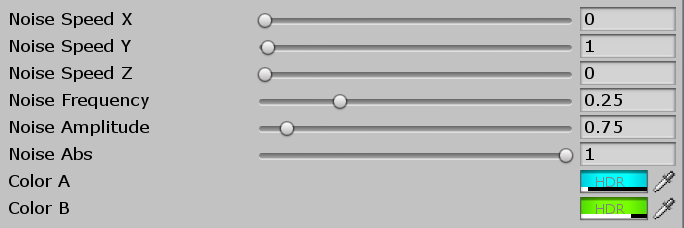### Particle System 2

This is the glowing red 3D wavebed.

[IMAGE REMOVED]

Set the transform as follows. Position XYZ = (0, -1.25, 8), Rotation XYZ = 0, Scale XYZ = (3, 0.5, 1).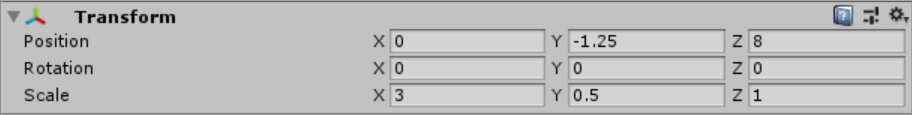Set the Render Mode to Mesh and then set the Mesh to Cube.The only changes to the material for this system are the colours. Both are red with alphas 0 and 25 respectively.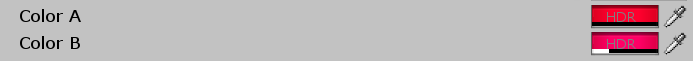### Particle System 3

This should be a duplicate of the previous sytem, because we’ll once again be using a cube mesh emitter to create a blue wavebed.

[IMAGE REMOVED]

Once again, we need to set the Transform properties. Position XYZ = (0, -3.15, 10). Rotation XYZ = 0. Scale = (12, 1, 1).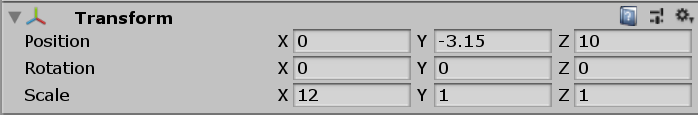Lastly, we just need to change the material colours to dark blue and aqua with opacities of 5 and 25 respectively.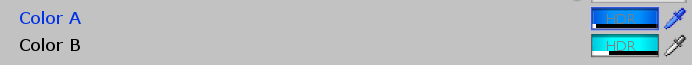And that’s everything! You should have something similar to the end effect now.

[IMAGE REMOVED]

## 4 thoughts on “GPU Particle Animation w/ Simplex Noise”

1. Thanks so much for these tutorials, I think there may be a typo in section 3, you say
“float noiseAbs = lerp(noise, noise01, _NoiseAbs);”
but I beleive that should be “float noiseRemap”

1. Hi David,

Regards,
– Mirza

2. Hey Mirza, thank you so much for this tutorial first of all!
I’m having some trouble with the “#include “SimplexNoise3D.hlsl” line. Unity doesn’t seem to know how to access the file?
I might just not be understanding what exactly an HLSL file is. Currently I have it saved in the Assets directory of my project. I’m not sure if this is correct? Any help would be much appreciated!

3. Hey Mirza, first I’d like to say thank you for the amazing tutorials!
I’m having some trouble with the #include “SimplexNoise3D.hlsl” line.

Unity is giving me the error:
Shader error in ‘Unlit/Simplex_Wave_Shader’: failed to open source file: ‘SimplexNoise3D.hlsl’ at line 25 (on d3d11)

I’m thinking I didn’t save the file to the right place, since right now it’s just sitting in my Assets directory. Any help would be much appreciated!